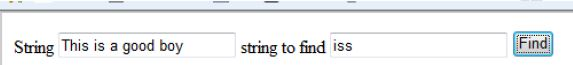# Finding characters in string in JavaScript

##### How to find characters in a given string in JavaScript?

In case we need to find characters in a given string using JavaScript, we can follow this approach.

```    String
<input type="text" name="txtName" id="txtName" value="This is a good boy" />
string to find
<input type="text" name="txtFind" id="txtFind" value="iss" />
<input type="button" name="btnTestfunction" value="Find" onclick="TestFunction()" />

<script>
function TestFunction() {
var string = document.getElementById("txtName").value;
var toFind = document.getElementById("txtFind").value;
var find = new RegExp(toFind);
}
</script>
```

In the above code snippet, we have two textboxes. The 1st textbox contains string and the 2nd textbox contains characters to find from the string.

Onclick of the button, we are calling “`TestFunction()`” function. In this function we are retrieving the value of textboxes and then instantiating the `RegExp` (Inbuilt in JavaScript) object by passing the string to find. Then calling the test method of `RegExp` object by passing the string from which we need to find characters.

OUTPUTViews: 3701 | Post Order: 9